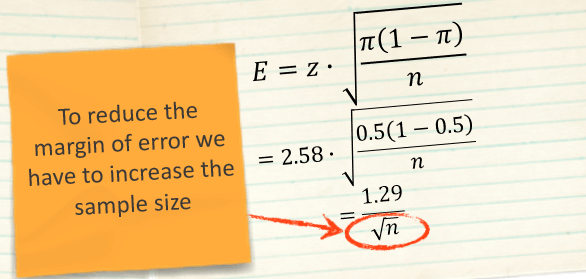# 4.4 Sample Size

### Determining the Sample Size

The sample size does not depend on the size of the population being studied, but rather it depends on qualitative factors of the research.

• desired precision of estimates
• knowledge of population parameters
• number of variables
• nature of the analysis
• importance of the decision
• incidence and completion rates
• resource constraints### Sample Sizes Used in Marketing Research Studies

 Type of Study Minimum Size Typical Size Problem identification research (e.g., market potential) 500 1,000 – 2,000 Problem solving research (e.g., pricing) 200 300 – 500 Product tests 200 300 – 500 Test-market studies 200 300 – 500 TV/Radio/Print advertising (per commercial ad tested) 150 200 – 300 Test-market audits 10 stores 10 – 20 stores Focus groups 6 groups 10 – 15 groups

### Margin of Error Approach to Determining Sample Size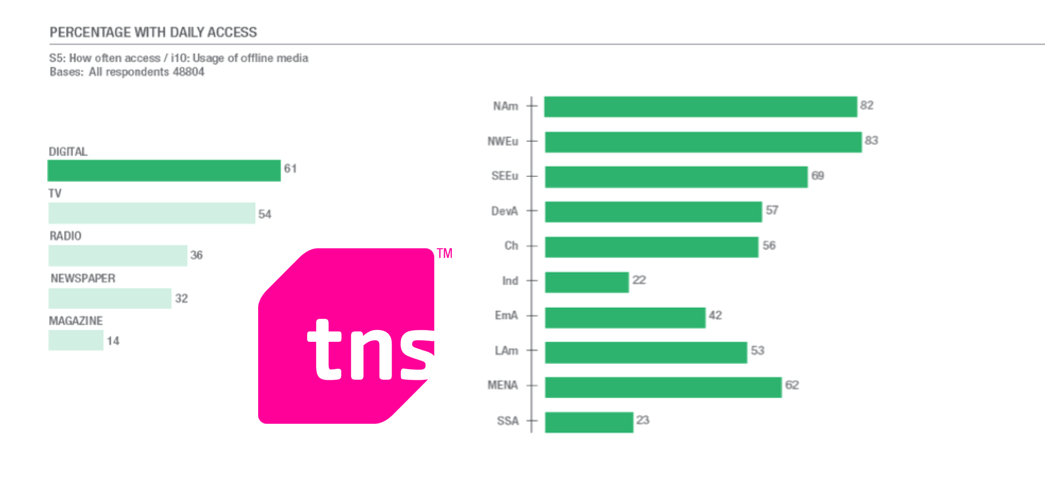### Margin of Error Approach to Determining Sample Size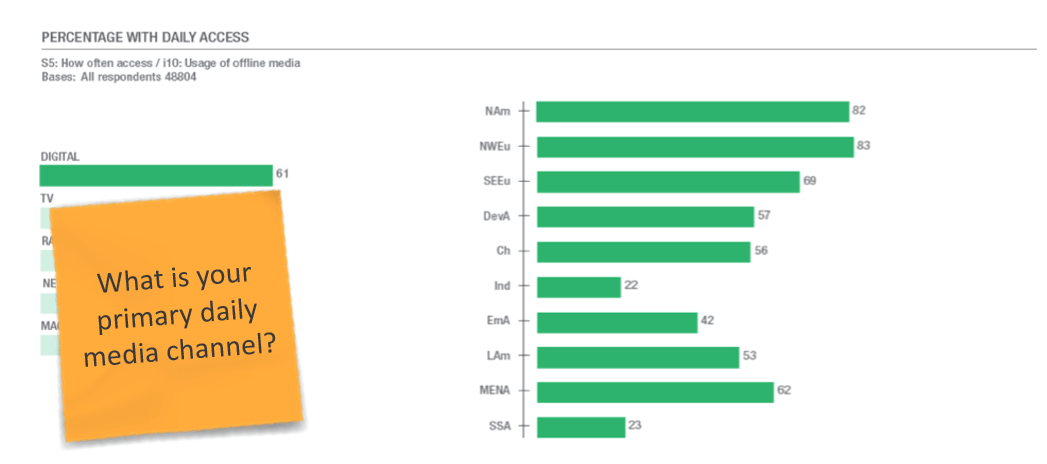The Margin of Error is the measure of accuracy of a survey. The smaller the margin of error, the more accurate are the estimates of a survey.

How accurate is this statistic? What is the margin of error?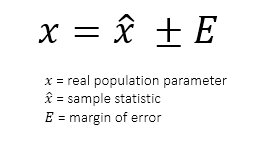Means

use this formula when evaluating estimates of population means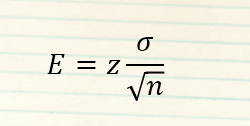z = z-value for a given level of confidence
σ = standard deviation of a population parameter
n = sample size

Proportions

use this when evaluating estimates of proportions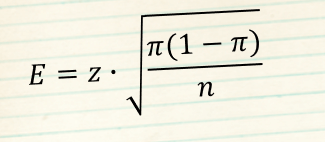z = z-value for a given level of confidence

π = estimate of the proportion in the population

n = sample size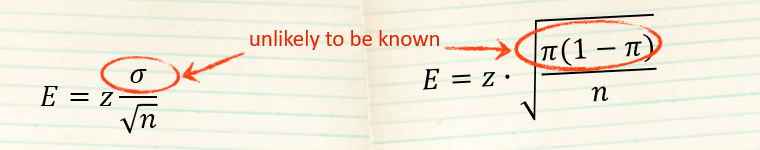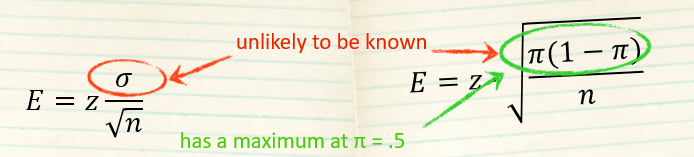z-values

z = 1.96

for 95% level of confidence

z = 2.58

for 99% level of confidence

maximum margin of error  for 95% level of confidence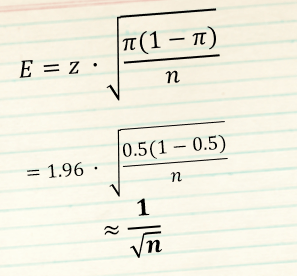### Margin of Error Approach to Determining Sample Size

How accurate is this statistic? What is the margin of error?

Margin of Error = 1/√n

48,804 people in sample

√48,804=220.916

1/221 = 0.0045

*100 = 0.45%

x = 61% ± 0.45%

60.55% to 61.45%

calculations are approximate values for 95% level of confidence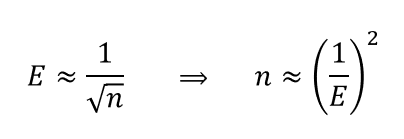How large should the sample be taking margin of error of ±1% into account?

Sample Size = (1/Margin of Error)2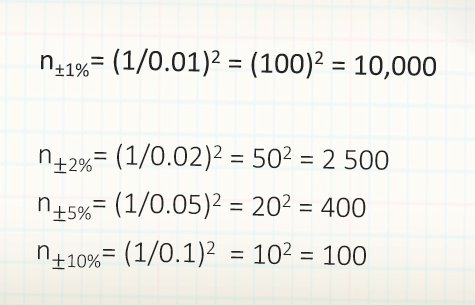calculations are approximate values for 95% level of confidence

## Corrections needed, when sample size exceeds 10% of the population

### Sample Size = (1/Margin of Error)2

Sample Size does not depend on population.

n±1%= (1/0.01)2 = (100)2 = 10,000

What if the population under study consists of only 100 elements? (e.g., firms producing cars)

Correction of the Sample Size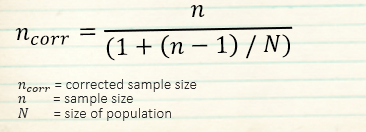Margin of Error 1%

What if the population under study consists of only 100 elements? (e.g., firms producing cars)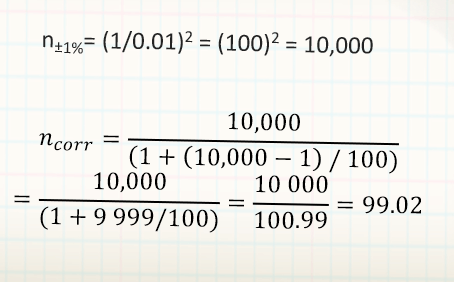Margin of Error 5%

What if the population under study consists of only 100 elements? (e.g., firms producing cars)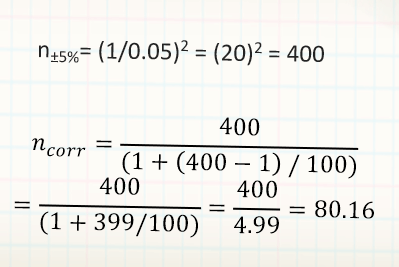Margin of Error 10%

What if the population under study consists of only 100 elements? (e.g., firms producing cars)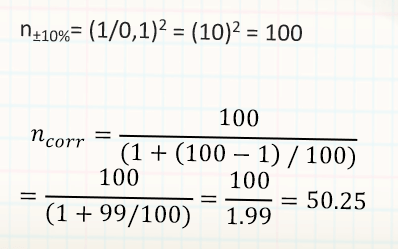### A Note on Confidence Interval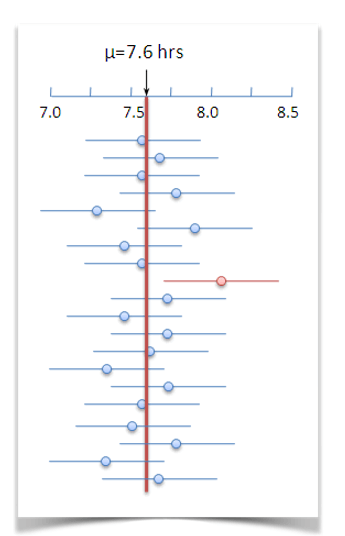Confidence Interval & Level of Confidence

A confidence interval estimate is an interval of numbers, along with a measure of the likelihood that the interval contains the unknown parameter.

The level of confidence is the expected proportion of intervals that will contain the parameter if a large number of samples is maintained.

Suppose we’re wondering what the average number of hours that people at Siemens spend working. We might take a sample of 30 individuals and find a sample mean of 7.5 hours. If we say that we’re 95% confident that the real mean is somewhere between 7.2 and 7.8, we’re saying that if we were to repeat this with new samples, and gave a margin of ±0.3 hours every time, our interval would contain the actual mean 95% of the time.

### Confidence Interval, Margin of Error, and Sample Size

The higher the confidence we need, the wider the confidence interval and the greater the margin of error will be

z-values

z = 1.96

for 95% level of confidence

z = 2.58

for 99% level of confidence

maximum margin of error for 99% level of confidence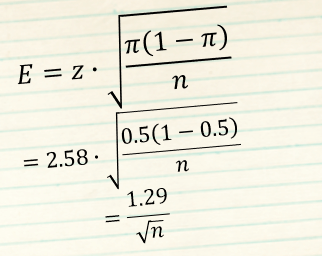smaller margins of error

require larger samples

higher levels of confidence

require larger samples